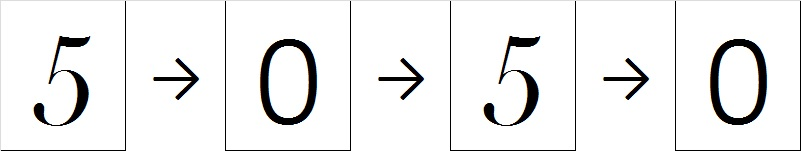# 279 How to Add and Subtract 5 with Secret Identities

• 279 is a composite number.
• Prime factorization: 279 = 3 x 3 x 31, which can be written (3^2) x 31
• The exponents in the prime factorization are 2 and 1. Adding one to each and multiplying we get (2 + 1)(1 + 1) = 3 x 2  = 6. Therefore 279 has 6 factors.
• Factors of 279: 1, 3, 9, 31, 93, 279
• Factor pairs: 279 = 1 x 279, 3 x 93, or 9 x 31
• Taking the factor pair with the largest square number factor, we get √279 = (√9)(√31) = 3√31 ≈ 16.703Do you know who Superman is? I’ve asked many children in their first year of school that question. They know who he is, and they can tell me lots of things about him.Superman’s secret identity is Clark Kent, but did you know that the numbers 1, 2, 3, 4, and 5 also have secret identities? Before today, hardly anybody has known what their secret identities are, but I will reveal them to you now!

Let’s start with five. Its secret identity is zero. Five can change into a zero by smoothing its top and curving it down. When you count by fives you can see it changing into zero and back into five again as fast as Superman can change into Clark Kent.  5, 10, 15, 20, 25, 30, 35, 40, 45, 50, 55, 60, 65, 70, 75, 80, 85, 90, 95, 100, 105, and so forth.By using its secret identity , we can easily add or subtract five from a number ending in five or zero! 5 + 5 = 10, 10 + 5 = 15, and 15 – 5 = 10; 10 – 5 = 5.

Have you ever noticed how easy it is to turn a 4 into a 9?

That was as easy as Superman becoming Clark Kent!

When you count by 5’s starting at 4, these numbers turn into each other over and over again: 4, 9, 14, 19, 24, 29, and so forth. When you add 5 to either 4 or 9, it will turn into the other: 4 + 5 = 9, 9 + 5 = 14. The same thing happens when you subtract five: 14 – 5 = 9; 9 – 5 = 4. There isn’t any reason to count up or down; just remember how easy it is to turn a 4 into a 9 and vice versa.

A 3 can easily turn into an 8:• Counting by 5’s starting at 3 we get: 3, 8, 13, 18, 23, 28, and so forth.
• Also 3 + 5 = 8; 8 + 5 = 13; 13 – 5 = 8; 8 – 5 = 3.

If you cut off the tail at the bottom of a two, it can easily turn into its secret identity, seven:• Counting by 5’s starting at 2, we get: 2, 7, 12, 17, 22, 27, and so forth.
• 2 + 5 = 7; 7 + 5 = 12 and 12 – 5 = 7; 7 – 5 = 2.

Here’s how to discover the secret identity for the number 1. Take a strip of paper that looks like the number 1 and follow these SAFE directions for curling ribbon (or paper) with scissors. Curl the bottom half of the number 1 as well as the top fourth of that number 1 with scissors to make that number 1 look just like the number 6.• Counting by 5’s starting at 1, we get: 1, 6, 11, 16, 21, 26, and so forth.
• 1 + 5 = 6; 6 + 5 = 11 and 11 – 5 = 6; 6 – 5 = 1.

When Superman puts on glasses and a suit, we see his secret identity. Did you notice that odd digits have even secret identities and even digits have odd secret identities? Once a child memorizes these digits and their secret identities, he or she will be SUPER at adding or subtracting 5 and will never need to count up or down to get the answer.

Using these number transformations can help children memorize other addition facts. Once they know how to Add 1, 2, 3 and 4 and how to add 5, they can use that information to add 6, 7, 8, 9, or 10 by breaking up those numbers into a smaller number plus five. For example:

• 6 + 7 = 6 + (2 + 5) = (6 + 2) + 5 = 8 + 5 = 13.
• 7 + 9 = 7 + (4 + 5) = (7 + 4) + 5 = 11 + 5 = 16.

Learning facts for 6, 7, 8, 9, and 10 this way isn’t any more complicated than the common core approach[6 + 7 = 6 + (6 + 1) = (6 + 6) + 1 = 12 + 1 = 13], but is perhaps not as easy as just memorizing those facts and using flash cards or other means to drill them permanently into the brain. Secret identities and even “Flash” cards can be super fun.